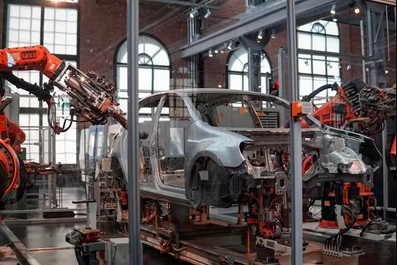A car company currently uses $2,000 hand tools for a portion of its prototype production. The hand tool process adds$1.75 in manufacturing costs per unit. The company wants to reinvest some profits to optimize the assembly line, and is looking to automate this specific hand tool section. A potential vendor quoted $20,000 for new machinery, with a projected manufacturing cost of$0.25 per unit. If the company were to upgrade to an automated system, what would be the anticipated payback period (in years) when the production floor historically produces 6,000 prototype units annually?Hint
The payback period is the period of time needed for the profit or other benefits from an investment to equal the cost of the initial investment.
Hint 2
First find the total annual manufacturing cost of the hand tool, then compare it to the total annual manufacturing cost of the automated system. The difference between these numbers is the annual savings. Divide the additional upfront investment by the annual savings to determine the pay back period.
By altering the value of any one situation’s variables, it’s possible to determine a factor for that variable that makes the two alternatives economically equal, assuming all other considerations are held constant. This value is the breakeven point. Breakeven analysis is utilized to describe an operation’s capacity percentage where income will only cover expenses. The payback period is the period of time needed for the profit or other benefits from an investment to equal the cost of the initial investment.

First, let’s find the total annual manufacturing cost of the hand tool, then compare it to the total annual manufacturing cost of the automated system to determine the annual savings.
($1.75)(6,000 units) =$10,500 in manufacturing costs/year
($0.25)(6,000 units) =$1,500 in automated manufacturing costs/year
Annual savings = $10,500 -$1,500 = $9,000 with automation To determine the payback period: Additional investment (on top of the original hand tools) =$20,000 - $2,000 =$18,000
Payback period = $18,000/$9,000 = 2 years
2 years